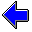Java Security Settings:
This web page employs Java, which requires specific security settings for correct operation.
If the applets on this page do not run correctly, consult the Virtual Chemistry Experiments FAQ
or the Physlet Physics web site for establishing the correct security settings.

# Gas Laws

## Charles's Law

### Concepts

The next significant advance in the study of gases came in the early 1800's in France. Hot air balloons were extremely popular at that time and scientists were eager to improve the performance of their balloons. Two prominent french scientists, Jacques Charles and Joseph-Louis Gay-Lussac, made detailed measurements on how the volume of a gas was affected by the temperature of the gas. Given the interest in hot air balloons at that time, it is easy to understand why these men should be interested in the temperature-volume relationship for a gas.

Just as Robert Boyle made efforts to keep all properties of the gas constant except for the pressure and volume, so Jacques Charles took care to keep all properties of the gas constant except for temperature and volume. The equipment used by Jacques Charles was similar to that employed by Robert Boyle. A quantity of gas was trapped in a J-shaped glass tube that was sealed at one end. This tube was immersed in a water bath; by changing the temperature of the water Charles was able to change the temperature of the gas. The pressure was held constant by adjusting the height of mercury so that the two columns of mercury had equal height, and thus the pressure was always equal to the atmospheric pressure.

Intuitively, it is expected that the volume of the gas will increase as the temperature increases. Is this relationship linear? A plot of V vs T can be used to test this hypothesis.

If a decrease in temperature results in a decrease in volume, what happens if the temperature is lowered to a point where the volume drops to zero? A negative volume is obviously impossible, so the temperature at which the volume drops to zero must, in some sense, be the lowest temperature that can be achieved. This temperature is called absolute zero.

### Experiment

Objectives

• Determine how the volume of a gas changes with the temperature for a fixed amount of gas and pressure.
• Determine absolute zero.

A sample of air is trapped in the closed end of the manometer. (The air has been given an artificial light green color to illustrate its presence.) The amount of mercury in the manometer has been adjusted so that the two columns of mercury have the same height, and thus the pressure of the gas equals the atmospheric pressure.

Carefully measure the height of the column of trapped air and determine the volume of the trapped gas. The inside diameter of the manometer tube is 4.286 cm. Read the temperature from the thermometer, enter the temperature and volume in the boxes provided, and plot the point on the graph.

Change the temperature of the system by dragging the liquid in the thermometer to a higher or lower level. (This is admittedly a somewhat fanciful way to alter the temperature.) The volume of the gas will change in response to the temperature change. Carefully adjust the amount of mercury in the manometer so that the heights of the two columns of mercury are identical. Then measure the height of the column of gas and calculate the volume of the gas. Continue this process until data is obtained at at least five different temperatures.

For each pair of volume-temperature values, enter the data in the table. The point will automatically be plotted on the graph along with the line-of-best-fit.

Is the plot of V vs T linear?

At what temperature does V = 0? This temperature is absolute zero. The absolute temperature is a temperature scale in which zero corresponds with absolute zero and the size of a degree is the same as the size of a degree in the celcius scale.

V = mL
T = oC

Temperature
( oC )
Volume
( mL )

Slope =           Intercept =Calculations using Boyle's Law                     Avogadro's LawGas Laws Home PageVirtual Chemistry Home Page

CharlesLaw.html version 2.1